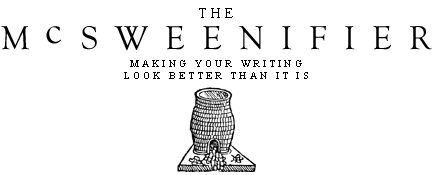```
```S E V E R A L   O C C U R A N C E S O F   T H E   T I T L E   O F   A S H O R T   S T O R Y   B Y R I C H A R D   M .S T A L L M A N , E A C H O C C U R A N C E   H A V I N G H A D   T H E   N A M E   O F   A C E R T A I N   F I L E   A C C E S S O P E R A T I O N   R E P L A C E D W I T H   T H E   N A M E   O F   A D I F F E R E N T   F I L E A C C E S S   O P E R A T I O N . BY LEONARD RICHARDSON - - - - The Right to Append The Right to Block on Input The Right to Open for Random Access The Right to Seek The Right to Truncate to Zero Length